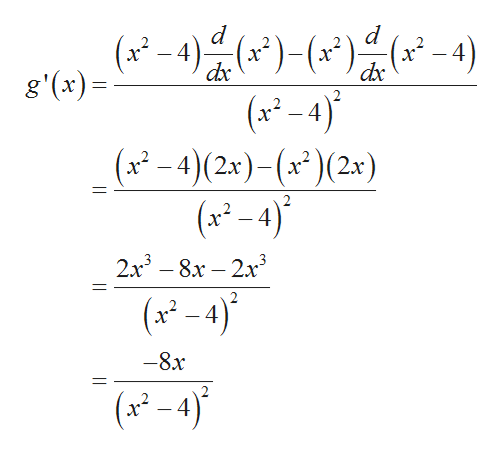# Consider the following function: g(x) = ((x^2)/(X^2)-4)Identify the following:d. Intervals where the function is increasing/decreasinge. Local extrema, give the location and value for any local maxima/minimaf. Concvity of the function and Points of Inflection (if they exist)

Question
1 views

Consider the following function:

g(x) = ((x^2)/(X^2)-4)

Identify the following:

d. Intervals where the function is increasing/decreasing

e. Local extrema, give the location and value for any local maxima/minima

f. Concvity of the function and Points of Inflection (if they exist)

check_circle

Step 1

According to the given information the given function is:

Step 2

For part (a)

It is required to calculate the interval where function is increasing or decreasing.

First find the first derivative of the function and equate it to zero to find the critical points.help_outlineImage Transcriptionclose-4)-4) (x-4) (x2-4)(2x)-(x )(2x) (x-4) d d dc dx g'(x) 2x38x-2x (x-4) -8x (x-4) fullscreen
Step 3

Now, put g&rsquo...

### Want to see the full answer?

See Solution

#### Want to see this answer and more?

Solutions are written by subject experts who are available 24/7. Questions are typically answered within 1 hour.*

See Solution
*Response times may vary by subject and question.
Tagged in

### Derivative